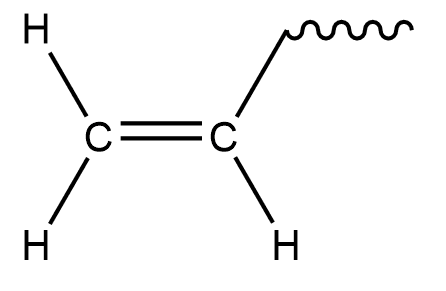QuestionAnswers

# Which of the following is a vinyl group?(A) $\left( {{\text{C}}{{\text{H}}_3}} \right){\text{CH}} -$(B) ${\text{HC}} \equiv {\text{C}} -$(C) ${{\text{H}}_2}{\text{C}} = {\text{CH}} - {\text{C}}{{\text{H}}_2} -$ (D) ${\text{C}}{{\text{H}}_2} = {\text{CH}} -$

Hint: We know that unsaturated hydrocarbons in which carbon atoms are bound to each other with double bonds are called alkenes. If one hydrogen atom from such compounds is removed, we can get a totally different compound.

Vinyl groups are also called ethenyl. It is basically ethylene with one less hydrogen atom less.
So, if we remove 1 Hydrogen from ethylene we get,Hence ${\text{C}}{{\text{H}}_2} = {\text{CH}} -$is a vinyl group.

So, the correct option is D.

Additional information: Vinyl groups need to be activated by some other group for enhanced reactivity. Activation increases the polarity of the bond and hence also increases reactivity. Vinyl groups are often found as intermediates in preparation of various organic compounds. In hydrohalogenation of alkynes i.e. (addition of hydrogen and halogen) vinyl groups containing intermediates are formed. For example –
$\begin{gathered} {\text{H}} - {\text{C}} \equiv {\text{C}} - {\text{H + H}} - {\text{Br }} \to {\text{ [C}}{{\text{H}}_2} = {\text{CH}} - {\text{Br] }} \to {\text{ C}}{{\text{H}}_3} - {\text{CH}} - {\text{B}}{{\text{r}}_2} \\ {\text{ethyne vinyl bromide dibromo ethane}} \\ \end{gathered}$
In the above reaction, when added to ethylene, vinyl intermediate is formed. Which on further reaction gives dibromo ethane. This reaction is used in preparation of secondary alkyl halides.
Vinyl groups are known to polymerize faster when a catalyst is used. Polymers formed are called vinyl polymers. However, these polymers do not contain any vinyl groups.
On industrial level manufacturing of polyvinyl chloride, vinyl chloride is used as a starting compound which contains vinyl groups.

Note: Out of the given four options, A is a tertiary butyl group and C option is an allylic group. Allylic group is often confused with vinyl group. We can remember it in a way that vinyl is formed when ethylene loses one hydrogen atom.